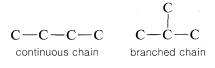# 2.4: Isomerization in Organic Compounds

$$\newcommand{\vecs}{\overset { \rightharpoonup} {\mathbf{#1}} }$$ $$\newcommand{\vecd}{\overset{-\!-\!\rightharpoonup}{\vphantom{a}\smash {#1}}}$$$$\newcommand{\id}{\mathrm{id}}$$ $$\newcommand{\Span}{\mathrm{span}}$$ $$\newcommand{\kernel}{\mathrm{null}\,}$$ $$\newcommand{\range}{\mathrm{range}\,}$$ $$\newcommand{\RealPart}{\mathrm{Re}}$$ $$\newcommand{\ImaginaryPart}{\mathrm{Im}}$$ $$\newcommand{\Argument}{\mathrm{Arg}}$$ $$\newcommand{\norm}{\| #1 \|}$$ $$\newcommand{\inner}{\langle #1, #2 \rangle}$$ $$\newcommand{\Span}{\mathrm{span}}$$ $$\newcommand{\id}{\mathrm{id}}$$ $$\newcommand{\Span}{\mathrm{span}}$$ $$\newcommand{\kernel}{\mathrm{null}\,}$$ $$\newcommand{\range}{\mathrm{range}\,}$$ $$\newcommand{\RealPart}{\mathrm{Re}}$$ $$\newcommand{\ImaginaryPart}{\mathrm{Im}}$$ $$\newcommand{\Argument}{\mathrm{Arg}}$$ $$\newcommand{\norm}{\| #1 \|}$$ $$\newcommand{\inner}{\langle #1, #2 \rangle}$$ $$\newcommand{\Span}{\mathrm{span}}$$$$\newcommand{\AA}{\unicode[.8,0]{x212B}}$$

More than one stable substance can correspond to a given molecular formula. Examples are butane and 2-methylpropane (isobutane), each of which has the molecular formula $$C_4H_{10}$$. Similarly, methoxymethane (dimethyl ether) and ethanol have the same formula, $$C_2H_6O$$:Compounds having the same number and kinds of atoms are called isomers.$$^2$$ Whereas only one stable substance is known corresponding to the formula $$CH_4$$, thirty-five stable isomers have been prepared of the formula $$C_9H_{20}$$. From this one may begin to sense the rich variety of organic chemistry, which leads to many problems - in telling one compound from another, in determining structures, and also in finding suitable names for compounds. In the rest of this chapter we will describe one type of isomer - the position isomer - and in later chapters we will discuss another type of isomer - the stereoisomer - and the experimental approaches that are used to establish the purity, identity, structure, and stereochemistry of organic compounds.

## Position Isomers

Compounds having the same number and kind of atoms but having different bonding arrangements between the atoms are called position isomers. Butane and 2-methylpropane are examples of position isomers. The atoms are connected differently in the two structures because the carbon chain in butane is a straight or continuous chain, whereas in 2-methylpropane it is branched:Therefore these two molecules are structurally different and, accordingly, do not have the same chemical and physical properties. They cannot be converted one into the other without breaking and remaking $$C-C$$ and $$C-H$$ bonds. Methoxymethane and ethanol are also position isomers because the oxygen clearly is connected differently in the two molecules:The term position isomer means the same as constitutional isomer. The designation structural isomer also is used, but this term is taken by some to include both position isomers and stereoisomers; that is, "structure" can mean both the way in which atoms are connected and their different arrangements in space.

The number of position isomers possible for a given formula rapidly increases with the increasing number of carbon atoms, as can be seen from the number of theoretically possible structures of formula $$C_nH_{2n+2}$$ up to $$n = 10$$ given in Table 2-3. In 1946, it was reported that all of the 75 compounds with values of $$n = 1$$ to $$n = 9$$ had been prepared in the laboratory. Before we can begin to discuss the chemistry of these compounds it is necessary to know howto name them; without convenient and systematic rules for nomenclature that are adopted universally, catastrophic confusion would result. We shall tackle this problem in the next chapter.

$$^2$$The prefix iso is from the Greek word meaning the same or alike.